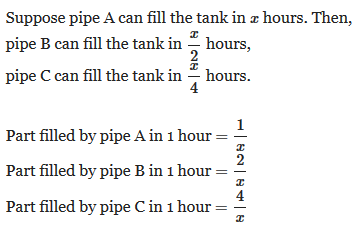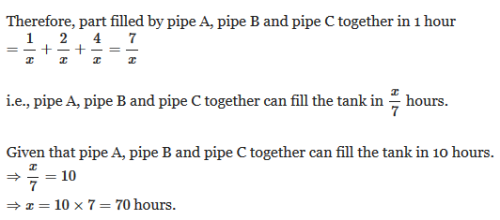Courses

# Test: Pipes And Cistern- 2

## 10 Questions MCQ Test General Aptitude for GATE 2020 | Test: Pipes And Cistern- 2

Description
This mock test of Test: Pipes And Cistern- 2 for LR helps you for every LR entrance exam. This contains 10 Multiple Choice Questions for LR Test: Pipes And Cistern- 2 (mcq) to study with solutions a complete question bank. The solved questions answers in this Test: Pipes And Cistern- 2 quiz give you a good mix of easy questions and tough questions. LR students definitely take this Test: Pipes And Cistern- 2 exercise for a better result in the exam. You can find other Test: Pipes And Cistern- 2 extra questions, long questions & short questions for LR on EduRev as well by searching above.
QUESTION: 1

### 1. Pipes A and B can fill a tank in 5 and 6 hours respectively. Pipe C can empty it in 12 hours. If all the three pipes are opened together, then the tank will be filled in:

Solution: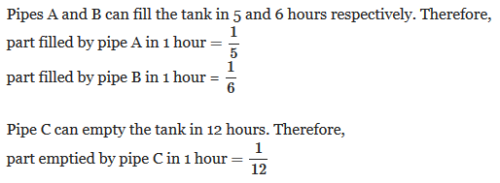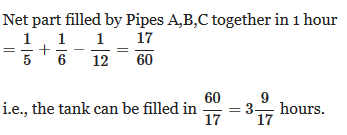QUESTION: 2

### Two pipes A and B can fill a cistern in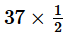minutes and 45 minutes respectively. Both pipes are opened. The cistern will be filled in just half an hour, if pipe B is turned off after

Solution: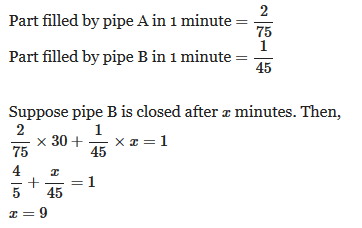QUESTION: 3

### A pump can fill a tank with water in 2 hours. Because of a leak, it took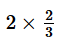hours to fill the tank. The leak can drain all the water of the tank in

Solution: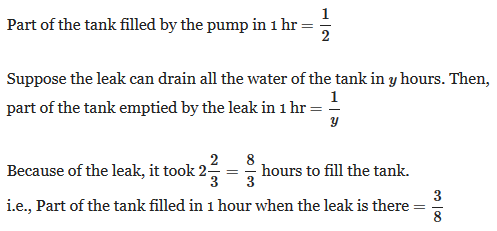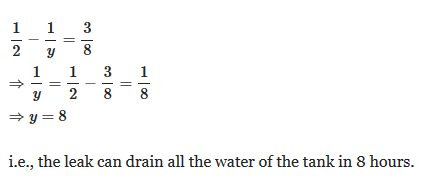QUESTION: 4

Three pipes A, B and C can fill a tank from empty to full in 30 minutes, 20 minutes, and 10 minutes respectively. When the tank is empty, all the three pipes are opened. A, B and C discharge chemical solutions P,Q and R respectively. What is the proportion of the solution R in the liquid in the tank after 3 minutes?

Solution:

Part of the tank filled by pipe A in 1 minute =1/30
Part of the tank filled by pipe B in 1 minute =1/20
Part of the tank filled by pipe C in 1 minute =1/10

Here we have to find the proportion of the solution R.

Pipe C discharges chemical solution R.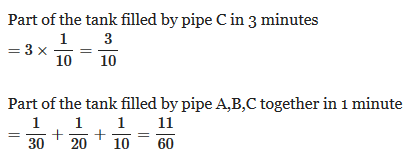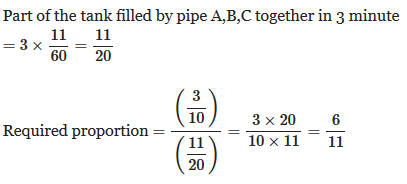QUESTION: 5

A tank is filled by three pipes with uniform flow. The first two pipes operating simultaneously fill the tank in the same time during which the tank is filled by the third pipe alone. The second pipe fills the tank 5 hours faster than the first pipe and 4 hours slower than the third pipe. Time required by the first pipe to fill the tank is

Solution: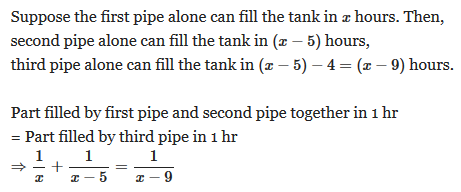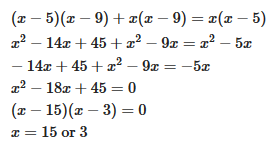We can not take the value x=3 because, (x−9) becomes negative which is not possible, because the third pipe can fill the tank in (x−9) hours.

Hence, x=15

QUESTION: 6

Two pipes A and B together can fill a cistern in 4 hours. Had they been opened separately, then B would have taken 6 hours more than A to fill the cistern. How much time will be taken by A to fill the cistern separately?

Solution: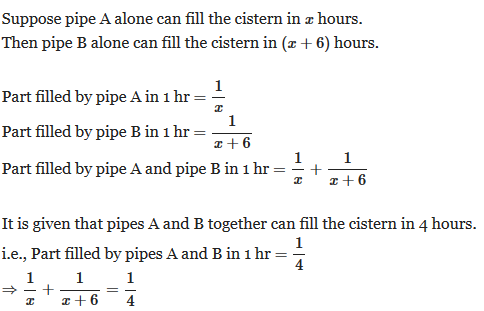QUESTION: 7

Two pipes A and B can fill a tank in 12 and 24 minutes respectively. If both the pipes are used together, then how long will it take to fill the tank?

Solution: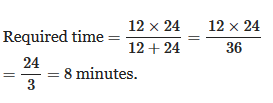QUESTION: 8

Two pipes A and B can fill a tank in 15 minutes and 40 minutes respectively. Both the pipes are opened together but after 4 minutes, pipe A is turned off. What is the total time required to fill the tank?

Solution: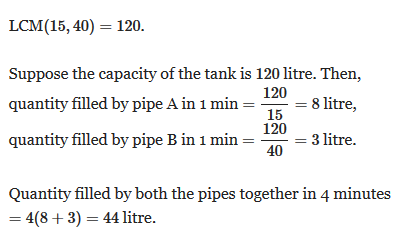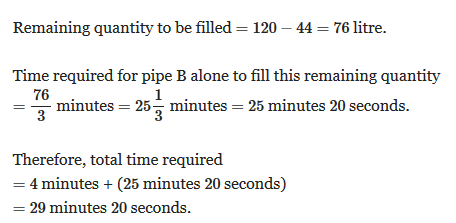QUESTION: 9

Two pipes can fill a tank in 25 and 30 minutes respectively and a waste pipe can empty 3 gallons per minute. All the three pipes working together can fill the tank in 15 minutes. The capacity of the tank is:

Solution: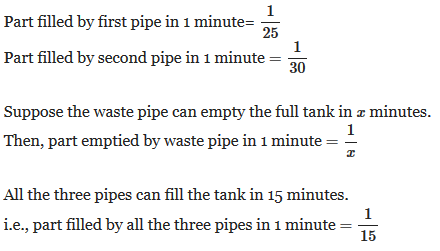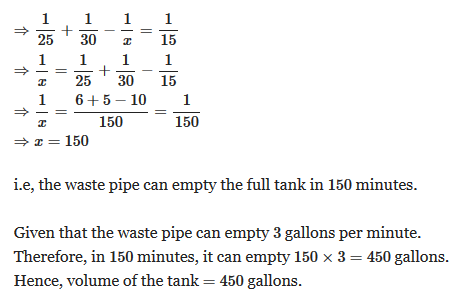QUESTION: 10

A tank is filled in 10 hours by three pipes A, B and C. Pipe C is twice as fast as B and B is twice as fast as A. How much time will pipe A alone take to fill the tank?

Solution: